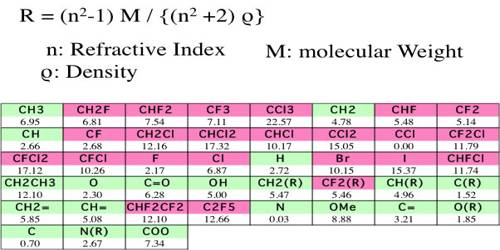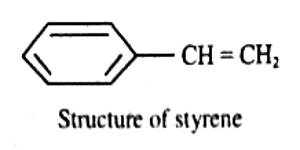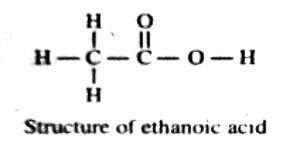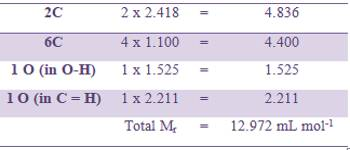Chemistry

# Molar RefractionMolar refraction has been found to be an additive as well as a constitutive property, and in the same way as for volume equivalent and parachor equivalent it has been possible to calculate the refraction equivalent of the elements and for structural factors like a double bond, triple bond etc. So, Molar Refraction is the equation for the refractive index of a compound modified by the compound’s molecular weight and density. Also known as the Lorentz-Lorenz molar refraction.

Table gives some refraction equivalent values.

Table: Refraction equivalents of elements and structural factors (Sodium-D line)Unlike parachor, ring formation has little effect on molar refraction. Although, in general, the molar refraction may be calculated with the help of refraction equivalents, some anomalies are observed, particularly with open chain compounds containing conjugated double bonds and with compounds in which the conjugated double bond is partly in the ring and partly in the side chain, as in styrene.Nevertheless, the refraction equivalents have been used in some cases in deciding between possible alternative structures of compounds. Let us take an example. The accepted structure of ethanoic acid has been shown to beThe molar refraction for ethanoic acid may be computed from the data presented in Table.The experimentally determined value of Mr for ethanoic acid is 13.300 mL mol-1. This is in good agreement with the calculated value. This confirms the structure of ethanoic acid as shown above.